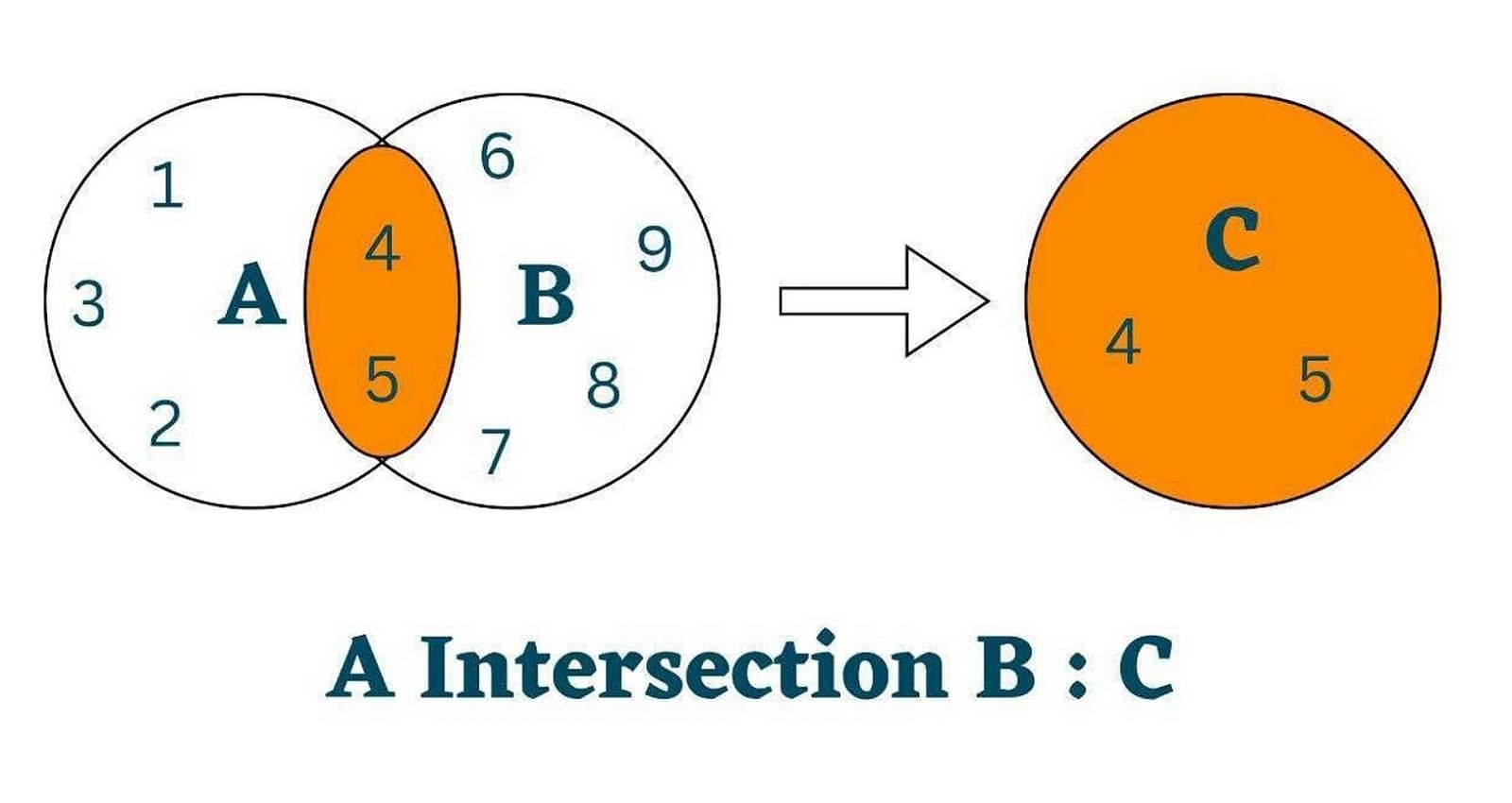# How to Find Intersection of Two Lists in Python? (with code)

A list is a robust and adaptable data structure in python that is frequently used to store and manage data collections. It offers a fantastic starting point for many programming tasks due to its simplicity and effectiveness. One important task we will learn here is how do you intersect two lists in Python.

But first, let's revise the basics of lists.

## What are lists?

Python has a built-in data type called lists that you may use to hold an ordered collection of objects. Object kinds that can be stored in lists include numbers, texts, and even other lists. Its entries are indexed such that they may be retrieved by position, starting at 0.

Lists are created by defining them with square brackets and a comma between each item. The values in a list can be of any data type, such as integers, floats, strings, or even other lists. For instance, the code below generates a list of integers:

```numbers = [1, 2, 3, 4, 5]
```

Lists can be utilized in a variety of ways. For instance, they can be combined with for loops to iterate through the list's items and carry out a specific operation on each one of them. They can also be utilized to implement queues and stacks of data.

## How do you intersect two lists in Python?

The intersection of two lists implies identifying the elements that are shared by both lists. Python provides numerous techniques to find this intersection.

Utilizing the built-in intersection() function from the collections module is the simplest way to accomplish this. The intersection() function returns a set that includes the elements that are shared by both lists.

Let's understand it will an example:

```import collections

list1 = [1, 2, 3, 4, 5]

list2 = [4, 5, 6, 7, 8, 9]

result = collections.Counter(list1) & collections.Counter(list2)

intersected_list = list(result.elements())

print(intersected_list)
```

Output:

```[4,5]
```

Understand it with this illustration:Using a for loop to cycle through the entries in one list and verify if they are present in the other list is another method for intersecting two lists. If so, you can include them in a new list that will serve as the intersection's result.

Here's the code for that:

```list1 = [1, 2, 3, 4, 5]

list2 = [4, 5, 6, 7, 8]

intersected_list = []

for element in list1:

if element in list2:

intersected_list.append(element)

print(intersected_list)
```

Output:

```[4,5]
```

Since each element in the other list must be verified, this method may be less effective for long lists. For beginners, it can be simpler to comprehend and offers greater control.

### Intersection of two lists without duplicates

Depending on the desired result, we can do the intersection of two lists in with or without duplicates.

If the same element appears in both lists when intersecting lists containing duplicates, the result may have numerous instances of that element. There are also situations where we may want no duplicates in the outcome when lists are intersected; each element will only appear once.

The method described in the previous explanation may be applied to intersect two lists of duplicates by using a for loop to cycle through one list and checking to see if each element is present in the second list. If so, it can be included in the list that is intersected. Here's the code:

```list1 = [1, 2, 3, 4, 5, 4, 5]

list2 = [4, 5, 6, 7, 8, 4, 5]

intersected_list = []

for element in list1:

if element in list2:

intersected_list.append(element)

print(intersected_list)
```

Output:

```[4,5,4,5]
```

In this example, the intersected list will contain [4, 5, 4, 5], since the duplicates are preserved.

You can use a set() to turn both lists into sets before using the intersection() function to identify the shared elements in two lists that do not contain duplicates. After that, the outcome can be changed back into a list.

Here is an example:

```list1 = [1, 2, 3, 4, 5, 4, 5]

list2 = [4, 5, 6, 7, 8, 4, 5]

set1 = set(list1)

set2 = set(list2)

intersected_set = set1.intersection(set2)

intersected_list = list(intersected_set)

print(intersected_list)
```

Output:

```[4,5]
```

In this example, the intersected list will contain [4, 5], with each element appearing only once.

### How to find the intersection of two lists of strings?

Similar to finding the intersection of two lists of integers or other data types, finding the intersection of two lists of strings in Python is also possible. The primary distinction is that == rather than it is used as the comparison operator for strings.

Here is an example of how to locate the intersection of two lists of strings using a for loop:

```list1 = ["apple", "banana", "cherry", "date"]

list2 = ["banana", "date", "elderberry", "fig"]

intersected_list = []

for element in list1:

if element in list2:

intersected_list.append(element)

print(intersected_list)
```

Output:

```["banana", "date"]
```

Banana and date are the items that are included in both list1 and list2, hence they will be present in the intersected list in this case.

Using the intersection() method from the set data structure is another method to determine the intersection of two lists of strings. Here's the code:

```list1 = ["apple", "banana", "cherry", "date"]

list2 = ["banana", "date", "elderberry", "fig"]

set1 = set(list1)

set2 = set(list2)

intersected_set = set1.intersection(set2)

intersected_list = list(intersected_set)

print(intersected_list)
```

Output:

```["banana", "date"]
```

In this example, the intersected list will again contain ["banana", "date"].

## Conclusion

To sum up, the built-in intersection() function from the collections module is the best way to find the intersection of two lists in python. We also learned how to intersect two lists without duplicates. Happy Learning :)

### FavTutor - 24x7 Live Coding Help from Expert Tutors!